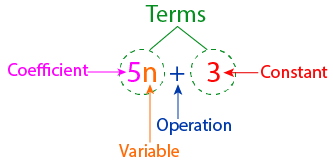Solving a linear equation

## Objective

To solve a linear equation, say 2x + 3 = 5

## What is an algebraic expression?

• Any expression involving a constant, a variable, and some operations like addition, multiplication, etc. is called an algebraic expression.
• A variable is an unknown number, and generally, it is represented by a letter like x, y, n, etc.
• Any number without any variables is called a constant.
• A number or quantity associated with a variable is called the coefficient of that variable.
• A term is any number or variable separated by operators.

Consider an expression like: 5n + 3

##What is linear equation?

• A linear equation is an equation in which the highest power of the variable is always 1. It could be in one variable or in two variables.
• The expressions that form the equation contain only one variable called a linear equation in one variable, i.e., the highest power of the variable appearing in the equation is 1.
• A linear equation in one variable is in the form of ax + b = 0, where a and b are any two integers and x is an unknown variable having only one solution.

### Solving a Linear Equation in One Variable

A value of the variable that, when substituted for the variable in an equation, makes L.H.S. = R.H.S. and thus satisfies the equation and is called a solution of the equation.

Some Important points related to Linear Equations

• There is an equality sign in the linear equation. The expression on the left of the equal sign is called the LHS (left-hand side) and the expression on the right of the equal sign is called the RHS (right-hand side).
• In the linear equation, the LHS is equal to RHS but this happens for some values only and these values are the solution of these linear equations.

Prerequisite Knowledge

Square

A square is a regular quadrilateral, which means that it has four equal sides and four equal angles of 90 degrees each.

Area of a Square

• The product of the length of each side itself.
• Formula : area of a square = side²

Rectangle

A rectangle is a four-sided quadrilateral with four right angles and opposite sides. sides equal. The length of a rectangle is the size of the longer side, whereas the breadth is the size of the shorter side.

Area of a Rectangle

• The product of its length and breadth
• Formula : area of a rectangle = length x breadth